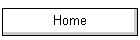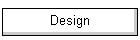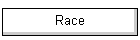I've been looking for an easy way to estimate depth of field. I want a single number that could estimate DoF for a lens, given focal length, aperture f, and subject distance, without too much error and without too much mental effort on the arithmetic.

We know that depth of field (DoF) depends on the focal length of the lens, distance to the subject, lens aperture, and the circle of confusion.  Wikipedia tells us the formula for the distance to the near DoF plane (where s = subject distance, L = lens focal length, f = lens aperture, and c = circle of confusion) is:Tricky to calculate this in your head. Photo Pills provides a handy chart on its Web site and a nice calculator app for your smartphone.  If you have an internet connection or have no time pressure, great.  On the other hand, sat at the table in the café wondering what aperture is needed to get a reasonably in-focus shot of my two companions as the birthday cake approaches, it is clear that none of this helps.

Putting aside the value of the circle of confusion, and given a specific focal length such as 17mm which is my “three-head”(*) prime lens, my idea was to have a simple parameter representing the depth of field at f/1 with the subject at 1 metre.  From there, I’d multiply up to the aperture I was using and multiply up to the actual subject distance.  Let’s imagine this parameter might have the value “80”, that is, DoF is 80 mm at f/1 with the subject at 1 metre.  Then DoF with the subject at 2 metres would be twice the parameter value, and at f4 would be four times.  Hey presto, I could quickly estimate the DoF for my two friends sat about 2 metres away with my lens set at f/4 — 2 ˣ 4 ˣ 80 = 640 mm.  That’s good, their heads are quite close together, but the light is pretty dim, could I get away with f/2 and keep ISO low?  Well, DoF would be around 320 mm here (2 ˣ 2 ˣ 80), so yeah, I’m in business….

(*) I like taking indoor and available-light snaps with friends and family, so have invested in the 45, 25, and 17 f/1.2 Oly lenses.  Turns out their use is best for one-, two-, and three-head scenarios.  They have also banished all thought of “full frame” since I no longer feel bokeh deprived, they perform like full-frame f/2.8 lenses.

## Near DoF Plane Only

Gazing at DoF tables quickly showed me that infinity cropped up pretty promptly if I wanted to use just one numeric value to estimate the overall “near to far” depth of focus.  This was because the far DoF plane would often run off to infinity with the sniff of a short lens or a stopped down aperture, while the near DoF plane never did.  So the first decision was that a single parameter should estimate the near part of overall DoF only, and doubling or trebling near DoF would easily cover the far part if the subject was not yet at the hyperfocal distance.  Let’s see if there is a parameter that will work with acceptable error.

This is a table of near DoF for a 17 mm MFT lens, that is, the DoF in front of the subject: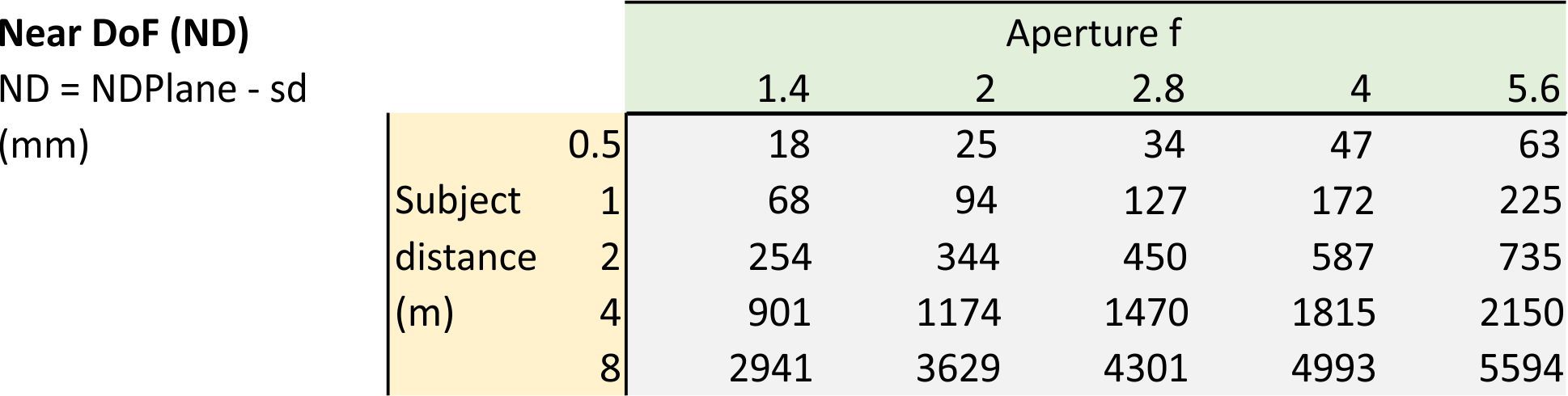The idea is to have a single parameter representing the depth of field that could apply to all apertures and all subject distances.  So if we divide the near DoFs by their aperture f and subject distance, we can see if there is any chance of a single number emerging.  The short answer is, no, not really.  But if we divide by subject distance squared, we can get reasonably close.  It turns out that near DoF is more or less linear in aperture f, and more or less quadratic in subject distance.

Given a single number parameter, NDp, the mental arithmetic needed therefore runs something like, "Square the subject distance, multiply by the aperture f, and multiply by NDp".  To ease the mental strain, and to make the estimates a little more accurate, the calculation involving subject distance can be split into two parts, using two numbers — the base NDp, and a 'subject distance adjustment', SDA. The mental arithmetic runs something like, "Multiply the SDA parameter value by the subject distance. Add this adjustment to the base NDp to give an adjusted 'single number parameter, NDp'. Multiply this adjusted parameter by the aperture f and by the subject distance again".

How much error is in the resulting near DoF estimate?  And second, what values of NDp and SDA minimise this error?

## Error

The first step is to define how we should measure error.  Let's use the example of an actual DoF of 94 mm (f/2, subject at 1 m) and imagine that a certain NDp and SDA suggest an estimated DoF of 160 mm.  The error is +66 mm, that is, an over-estimate of the true DoF.  For a different scenario, let's use the example of an actual DoF of 1815 mm (f/4, 4 m) and imagine the estimated DoF was 1881 mm.  The error is still +66 mm, again an over-estimate of the true DoF.  But it is clear that getting DoF wrong by 66 mm when it is actually 94 mm is quite an error compared to getting it wrong by 66 mm when it is actually 1815 mm.  Hence, an error is best expressed relative to some expected or actual value.  An error of 66 compared to 94 is a large 70% relative error, but compared to 1815 it is a small 3.6% relative error.  So know that we are going to use relative error as our measure of goodness of our estimates.

If the error of 66 mm is compared to the true value of 94 mm, the relative error is 70% — 66/94.  On the other hand, the error of 66 mm could be compared to the estimated DoF, in which case the relative error would be 41% — 66/160.  That seems quite a lot smaller, almost half the relative error.  In a different scenario, let's imagine that some NDp and SDA values suggest an estimate of DoF of 28 mm instead.  The error here is -66 mm, that is, an under-estimate of the true DoF.  If this error is compared to the true DoF, the relative error is -70% — -66/94.  On the other hand, the error of -66 mm could be compared to the estimated DoF, in which case the relative error would be -236% — -66/28.  In all four cases, the absolute amount of error is the same, 66 mm, but when compared relative to the true or to the estimated value, and keeping the sign as to under- or over-estimate, we get four different error values, -236%, -70%, +41%, and +70%.  Which values do we want to use to represent the error?

Usually, relative error is defined to be a comparison of the amount of error relative to the true value.  But as the above examples show, under-estimates can never be worse than -100% of the true value, while over-estimates can be 1000% or 10000% of the true value.  Usually, this isn't a problem, but with DoF, our preference might be different.  Imagine true DoF in a certain situation is 100 mm.  Then imagine the consequence if the NDp and SDA parameters suggested DoF to be 20 mm (an under-estimate of 80%) and on that basis took the shot because 20 mm DoF was what we wanted, compared to the consequence if we estimated DoF to be 180 mm (an over-estimate of 80%) and on that basis took the shot because 180 mm DoF was what we wanted.  In both cases, the subject at the specified distance is exactly in focus;  the issue is where the blur and bokeh begins.  With the under-estimate we'd have much reduced bokeh, much more of the shot in focus, and it would be nowhere near the artistic result we were looking for.  With the over-estimate we'd have somewhat less of the shot in focus and a little more bokeh, but it would not be that far away from the artistic result we wanted.  The 80% error of the under-estimate was a far worse error than the same 80% error of the over-estimate.  For this reason, we are going to use a relative error that is relative to the smaller of the two values of true or estimated DoF.  With this approach, we can get under-estimate errors of -1000% or -10000% just like over-estimate errors of +1000% or +10000%.

## Acceptable Error

Do we want to avoid large errors while accepting many small errors, or are some large errors acceptable provided most of the errors are very small? A common and popular way to measure average error is RMS (root mean square).  It has two characteristics.  First, RMS ignores the direction of the error, that is, whether it is an under- or over-estimate (because the error is squared and then processed).  Second, RMS gives more emphasis to larger error values, and much less emphasis to smaller errors (again, because the error is squared).  We will choose NDp and SDA parameter values that minimise RMS error, the usual procedure for avoiding large errors at the cost of accepting many smaller errors.

Do we want the errors to be balanced, that is, the under-estimate errors to be similar to the over-estimate errors?  Or do we want parameters whose error distribution is biased in a particular way?  Given our unusual definition of relative error, we've made sure that under-estimates give similar error values to over-estimates, so the RMS procedure should give us well-balanced errors.

## Calculating NDp and SDA

We want NDp and SDA values which minimise RMS error. We'll set up an Excel spreadsheet which does the arithmetic for us, and then try various NDp and SDA values by hand until we get to those which work. Or we can have the 'Solver' add-in do the goal-seeking calculations for us.

Here is a table of resulting 'best' NDp and SDA values for various lenses under various use case combinations with minimum RMS error.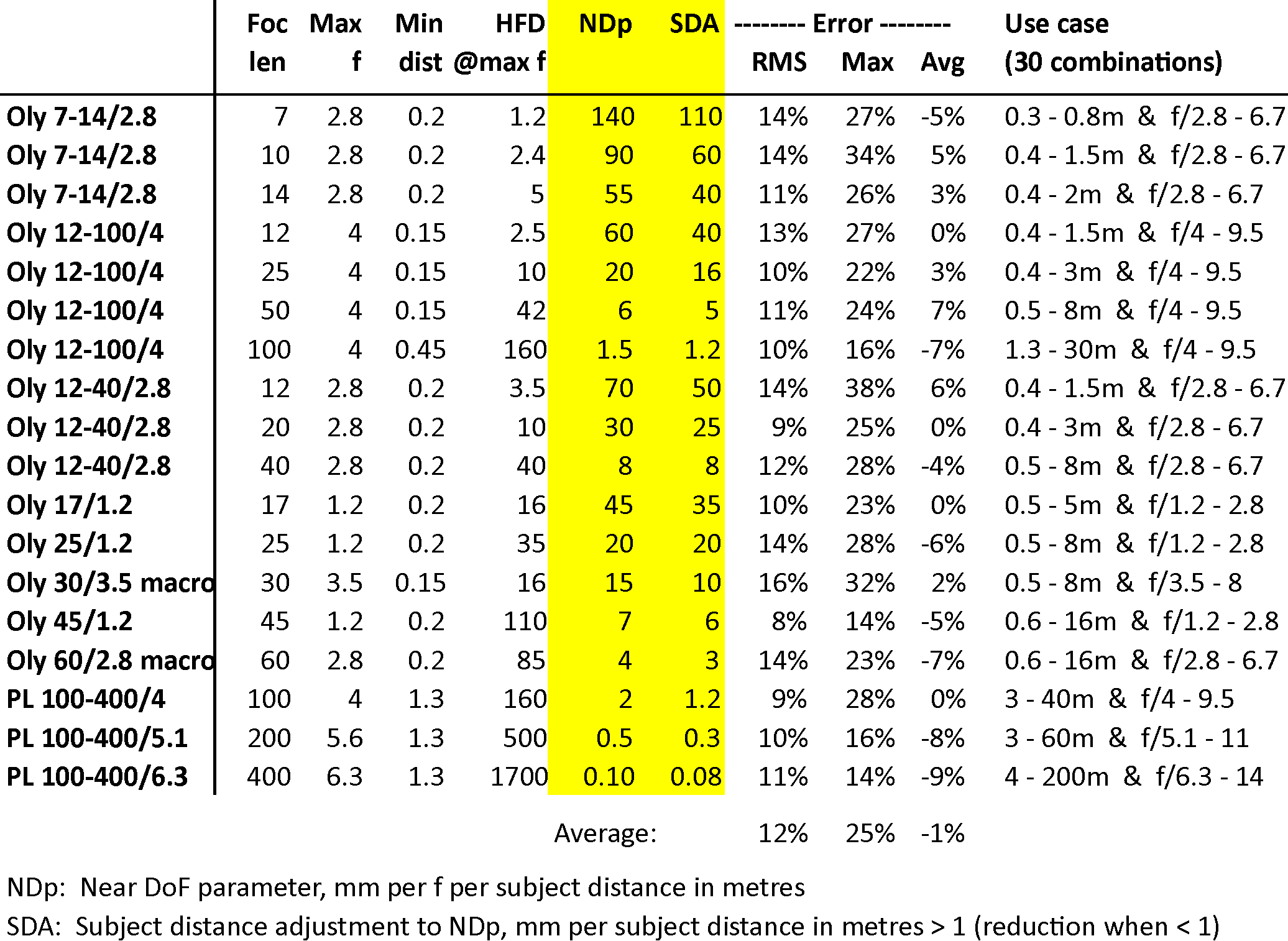## Using NDp and SDA

Taking the example of the Oly 17/1.2 lens, the table tells us that NDp = 45 and SDA = 35;  that these values yield 10% RMS error, with a worst case maximum error of 23%;  that, on average, under-estimates are exactly balanced by over-estimates (average error 0%);  and most importantly, that these results apply to subjects between 0.5 m and 5 m distant, and to aperture f values between f/1.2 and f/2.8.  So, I'm sat at the table watching the birthday cake approach.  My companions are 2 m away and the lens is at f/2.  What is the near DoF estimate?  The adjustment to NDp for subject distance is (2-1) ˣ 35 = 35 mm, so NDp' is 45 + 35 = 80.  The f value is 2 and the subject distance is 2, so estimated near DoF is 80 ˣ 2 ˣ 2 = 320 mm.  (Actually, near DoF here is 344 mm.)  Pretty good, I'm in business!

For interest, these are the tables of near DoF estimates, and relative errors, for the 17/1.2 using NDp = 45 and SDA = 35 for the "0.5 - 5 m & f/1.2 - 2.8" use case.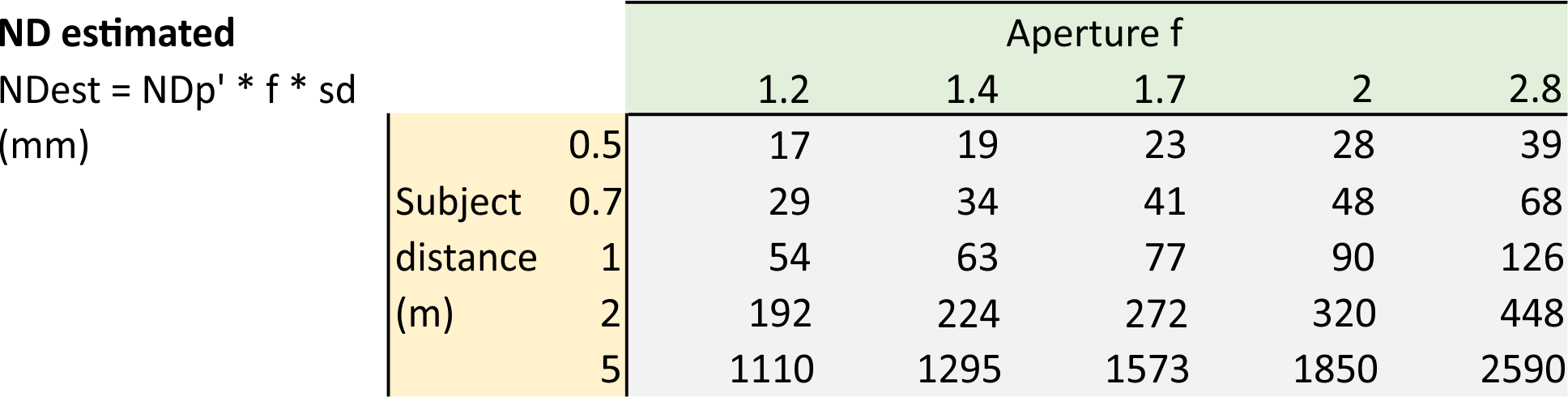## Remembering NDp and SDA

I don't want to remember the NDp and SDA values for every lens and every focal length, so I stick them to the lens hood.  Here is the relevant table stuck onto my Oly 12-100/4 lens hood.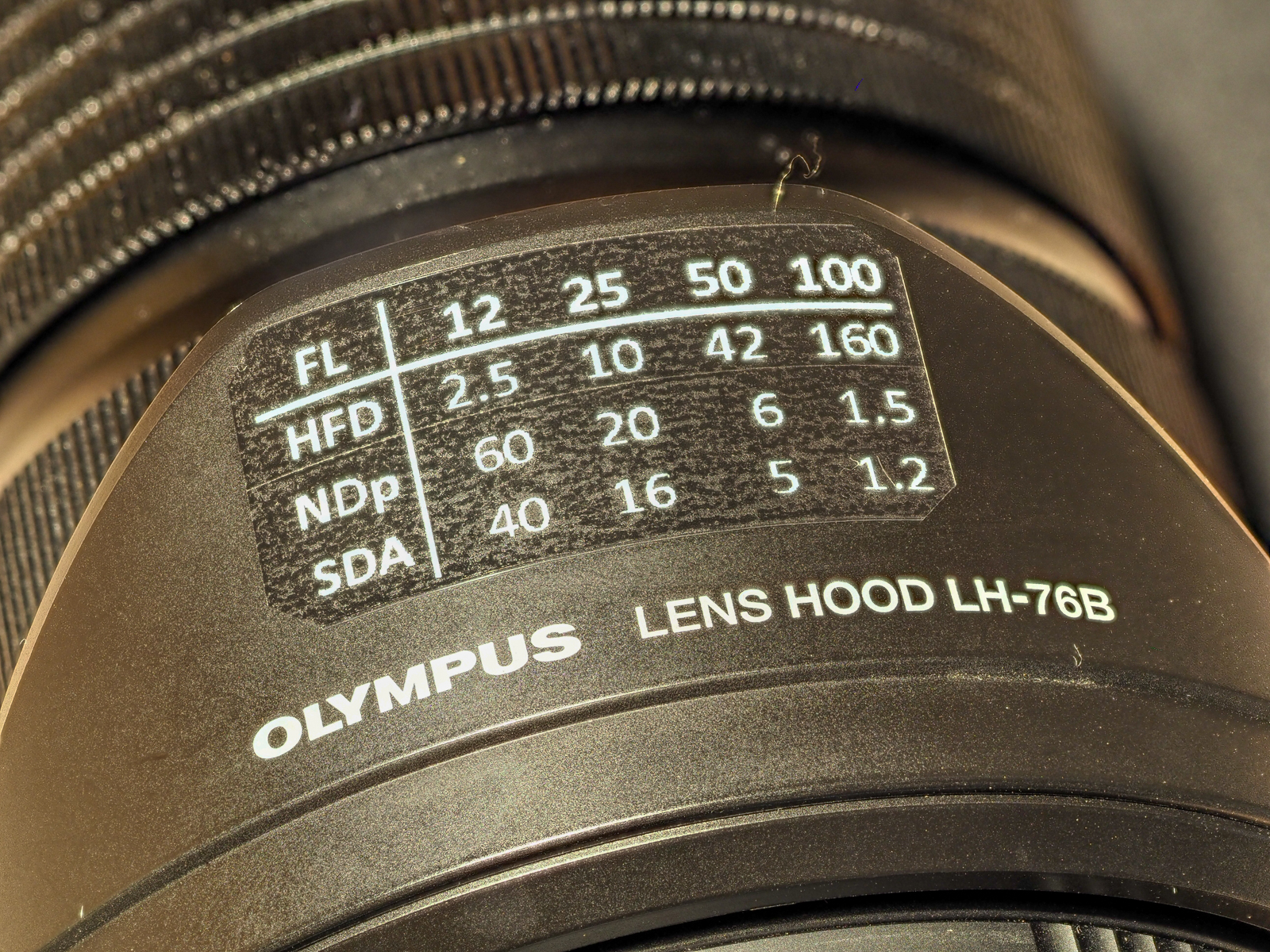The table also makes a note of 'HFD' — hyperfocal distance at maximum f — so I know when bokeh disappears even though I'm maxed out with a wide open aperture.

## Final note on being lazy

The optimum NDp and SDA values are similar.  For the 12-100/4 at 50 mm, for example, they are 6 and 5 respectively.  Under real pressure, or if I just can't be bothered, I'll use the easier of the two numbers, or their rough average.  So at 12 mm, for example, I'll use "50", the average of 60 and 40, or at 25 mm I'll just run with 20, an "easy" number for mental arithmetic.

In this case, the mental arithmetic is, "Multiply the parameter value by the aperture f and then by the subject distance twice (i.e. squared)."

Using the lazy "50" for FL = 12 mm, at f/4 with the subject at 1.2 m, I'd estimate near DoF to be 288 mm instead of the better estimate of 326 mm (actual near DoF is 400 mm), while using the lazy "20" for FL = 25 mm, at f/4 with the subject at 1.2 m, I'd estimate near DoF to be 115 mm instead of the better estimate of 111 mm (actual near DoF is 124 mm).  Heck, that'll do nicely, thanks!

It turns out that I can be quite lazy with the aperture f as well.  If f is anywhere near 4, such as f/5.6 or f/6.7, I'll use 4.  If it's over that, such as f/8 or f/11, I'll use a nice easy 10.

But I can't be too lazy with subject distance, that really does need as good a guess as possible, mainly because it is going to be squared.  For example, at FL = 25 mm and f/4 with the subject at 1.4 m, actual near DoF is 166 mm.  If I guessed the subject at 1 m, I'd estimate near DoF to be 80 mm, while if I guessed 2 m, the estimate would be 320 mm (using the lazy "20" parameter).